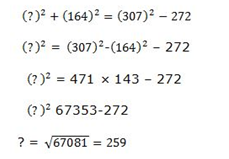# SBI Clerk Prelims 2021 Quantitative Aptitude Questions (Day-62)

Dear Aspirants, Our IBPS Guide team is providing new series of Quantitative Aptitude Questions for SBI Clerk Prelims 2020 so the aspirants can practice it on a daily basis. These questions are framed by our skilled experts after understanding your needs thoroughly. Aspirants can practice these new series questions daily to familiarize with the exact exam pattern and make your preparation effective.

Start Quiz

Partnership

1) A and B together can start the business with the investment of Rs.5000 and Rs.8000 respectively. After 6 months, C joined with them the investment of Rs.9000. At the end of one year the difference between the profits shares of A and C is Rs.200, what is the total profit share?

A.Rs.4000

B.Rs.6000

C.Rs.7000

D.Rs.5000

E.None of these

Trains

2) If the ratio of the length of train A to B is 3: 2 and the ratio of the speed of train A and B is 4: 5. If the time taken by train A crosses B running in opposite direction and same direction in 20 seconds and 180 seconds respectively, what is the speed of train A?

A.40 kmph

B.80 kmph

C.20 kmph

D.Cannot be determined

E.None of these

Average

3) Average age of A, B, C, D and E is 70 years and the average age of A , B and C,D is 70 years and 75 years respectively. What is E’s age after 10 years?

A.80 years

B.70 years

C.60 years

D.90 years

E.None of these

Boat and stream

4) A boat row along current and cover 20 km in one hour and the boat row against current and cover 30 km in 3 hours. What is the speed of the boat in still water?

A.10 kmph

B.15 kmph

C.20 kmph

D.25 kmph

E.None of these

Time And work

5) A can do the piece of work in 20 days and A and C together can complete the work in 15 days. If A, B and C together can complete the work in 10 days and they are gets the total wages is Rs.6600, then what is the wage of C?

A.Rs.1100

B.Rs.2200

C.Rs.3300

D.Rs.4400

E.None of these

Simplification

Directions (6-10): what will come in place of question mark (?) in the given questions?

6) 3√175616 × √1936 + (36)2 =?

A.3760

B.3860

C.3764

D.3770

E.None of these

7) (1.6)2 ÷ (0.8)2= [(2.4)2 ÷ (0.4)2] – ?

A.24

B.32

C.40

D.36

E.28

8) (3 / 5) of  (2 / 7)  of  (35 / 18) of ? = 405

A.1375

B.1275

C.1285

D.1215

E.1325

9) (?)
2+ (164) 2= (307) 2– 272

A.151

B.189

C.211

D.259

E.None of these

10) 24 % of 6550 – 175 % of ? = 697

A.500

B.475

C.675

D.825

E.975

Profit ratio = 5000 * 12: 8000 * 12: 9000 * 6

= 60: 96: 54

=10: 16: 9

Total profit = 35/1 * 200 = 7000

Distance = speed * Time

5x = 9y * 5/18 * 20

5x = 50y

x = 10y

5x = y * 5/18 * 180

5x = 50y

x = 10y

A + B+ C + D + E = 70 * 5=350

A + B = 70 * 2 = 140

C + D = 75 * 2 = 150

E = 350 – 140 – 150 = 60

E’s age after 10 years = 60 + 10 = 70 years

Speed of the boat in still water = x

Speed of the stream = y

Downstream speed = x + y

x + y = 20/1 = 20 kmph

Upstream speed = x – y

x – y = 30/3 = 10 kmph

Speed of the boat in still water = 1/2(downstream speed + upstream speed)

= 1/2 * (20 + 10)

= 15 kmph

Work done by A in one day,

A = 1/20

Work done by A and C in one day,

A + C = 1/15

Work done by C in one day,

C = 1/15 – 1/20

C = 1/60

Work done by A, B and C in one day,

A + B + C = 1/10

1/20 + 1/60 + B = 1/10

B = 1/10 – 1/20 – 1/60

Work done by B in one day,

B = 1/30

Ratio of work done by A, B and C = 1/20 : 1/30 : 1/60

= 3: 2: 1

C’s wage = 1/6 * 6600 = 1100

? = 3√175616 × √1936 + (36)2 =?

= 56 x 44 + 1296 = 2464 + 1296 = 3760

(1.6)2 ÷ (0.8)2 = [(2.4)2÷ (0.4)2] – ?

Or, (1.6×1.6) / (0.8×0.8) = [(2.4 x2.4) / (0.4×0.4)] – ?

or, 4 = 36-?

or, ? = 36 – 4 = 32

(3 / 5) × (2 / 7) × (35 / 18) × ? = 405

Or, ? = [(405 × 5 × 7 ×18) / (3 × 2 × 35)] = 121524 % of 6550 – 175 % of? = 697

Or, [(24 × 6550) / 100] – [175 ×?/ 100] = 697

or, [(1572 – 697) × 100] / 175 = ?

:. ? = (87500 / 175) = 500

 Check Here to View SBI Clerk Prelims 2021 Quantitative Aptitude Questions Day – 61 Day – 60 Day – 59